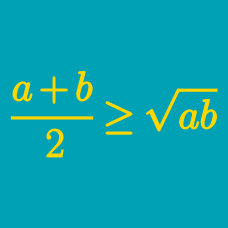Algebra

# Power Mean Inequalities: Level 2 Challenges

Let $x$ and $y$ be positive reals satisfying $x^2 + y^2 = 18$, find the maximum of $x + y$.

$\dfrac{a}{b} + \dfrac{b}{c} + \dfrac{c}{d} + \dfrac{d}{a}$

If $a, b, c$ and $d$ are any four positive real numbers, then find the minimum value of the expression above.

Given that $a,$ $b,$ and $c$ are positive reals such that $a+b+c=6$, find the maximum possible value of $abc$.

Find the minimum value of ${ a }^{ 2 }+{ b }^{ 2 }+{ c }^{ 2 }$ for positive numbers $a,b,c$ satisfying the constraint $a+b+c=6$.

For $a,b,c>0$ and $a+b+c=6$. Find the minimum value of

$\large \left (a+\frac{1}{b} \right )^{2}+ \left (b+\frac{1}{c} \right )^{2}+\left (c+\frac{1}{a} \right )^{2}$

Let $x,y$ be positive reals such that $x+y =2$, find the maximum value of $x^{3}y^{3}(x^{3}+y^{3})$.

×

Problem Loading...

Note Loading...

Set Loading...